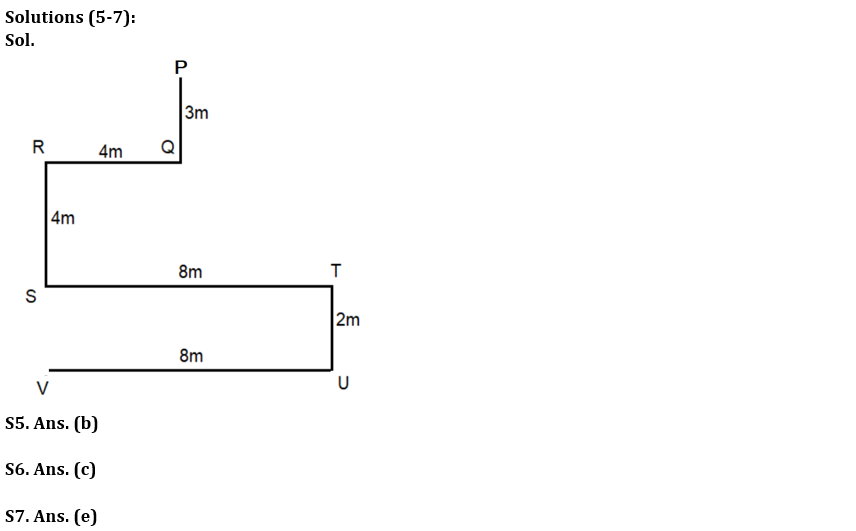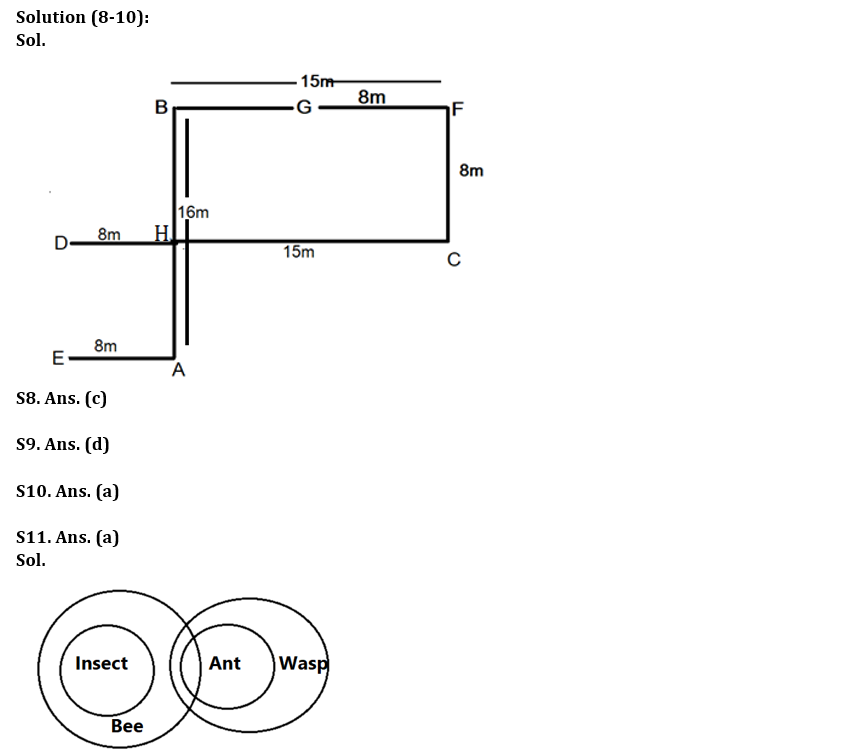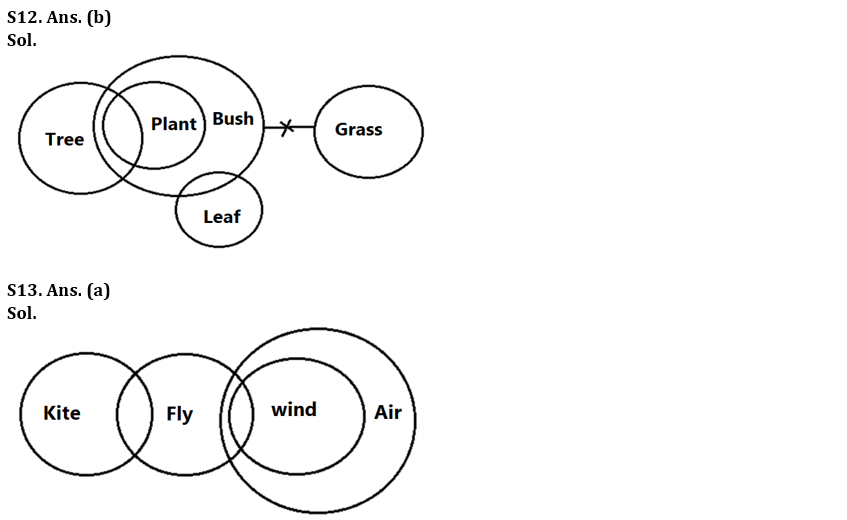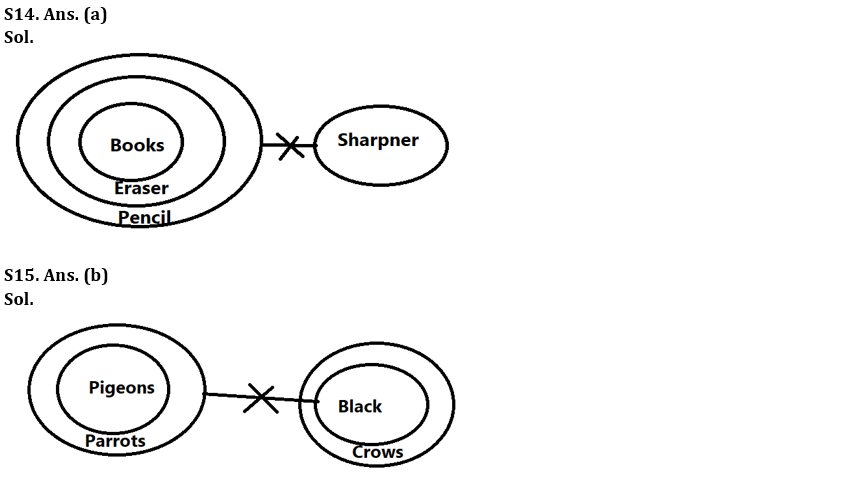Latest Banking jobs   »   Reasoning Ability Quiz For Bank Foundation...

# Reasoning Ability Quiz For Bank Foundation 2023 – 09th April

Directions (1-2): Answer the questions based on the information given below.
Six persons U, V, W, X, Y and Z have different weights. X is heavier than at least two persons. V is lighter than only one person. Z is heavier than Y who is heavier than W. U is heavier than X but not the heaviest.

Q1. How many persons are lighter than X?
(a) Three
(b) Four
(c) Five
(d) Two
(e) None of these

Q2. Who among the following person is 3rd heaviest?
(a) X
(b) Y
(c) U
(d) W
(e) Z

Directions (3-4): Answer the questions based on the information given below.
Six children (A, B, C, D, E and F) have different number of vehicles. E has more vehicles than only two persons. A has more vehicles than B and C but less than F. D has more vehicles than A. B does not have lowest number of vehicles. D does not have highest number of vehicles.

Q3. Which child has the 2nd highest number of vehicles?
(a) F
(b) D
(c) A
(d) E
(e) None of these

Q4. How many children are there in between D and C?
(a) Three
(b) Four
(c) One
(d) Two
(e) None of these

Directions (5-7): Answer the questions based on the information given below.
Deepak starts walking from point P in south direction. After walking 3m he reaches at point Q. He turns right from point Q and walks for 4m till point R. He then turns left from point R and walks for 4m till point S and then turns left from point S and walks for 8m till point T. He then turns right from point T and walk for 2m till point U and then turns right from point U, walks for 8m till point V and stops.

Q5. In which direction is point V with respect to point T?
(a) South
(b) South-west
(c) North
(d) North-east
(e) North-west

Q6. What is the shortest distance between point R and point V?
(a) 10m
(b) 14m
(c) 6m
(d) 8m
(e) 12m

Q7. In which direction is point R with respect to point U?
(a) South
(b) South-west
(c) North
(d) North-east
(e) North-west

Directions (8-10): Answer the questions based on the information given below.
Eight points A, B, C, D, E, F, G and H are drawn on paper. G is 8m west of F which is 15m east of B. B is 16m north of A which is 8m east of E. H is 15m west of C which is 8m south of F. H is 8m east of D.

Q8. What is the direction of F with respect to E?
(a) North-west
(b) South-west
(c) North-east
(d) South-east
(e) None of the above

Q9. What is the shortest distance between D and E?
(a) 5m
(b) 6m
(c) 7m
(d) 8m
(e) None of the above

Q10. What is the direction of G with respect to C?
(a) North -west
(b) South -west
(c) North -east
(d) South-east
(e) None of the above

Directions (11-15): In the question below, three statements are given followed by the conclusions. You have to take the given statements to be true even if they seem to be at variance with commonly known facts. Read all the conclusions and then decide which of the given conclusions logically follows from the given statements disregarding commonly known facts.

Q11. Statements: Only bee is insects.
Some bees are ants.
Each ant is wasp.
Conclusions: I. All insects are bees.
II. Some wasp is insects.
(a) Only conclusion I follows
(b) Only conclusion II follows
(c) Either conclusion I or II follows
(d) Both conclusions I and II follow
(e) Neither conclusion I nor II follows

Q12. Statements: Some plants are trees.
All plants are bush.
No bush is grass.
Some bush is leaf.
Conclusions: I. Some tree is leaf.
II. No plant is grass
(a) Only conclusion I follows
(b) Only conclusion II follows
(c) Either conclusion I or II follows
(d) Both conclusions I and II follow
(e) Neither conclusion I nor II follows

Q13. Statements: Some kite are fly.
Some fly are wind.
All wind are air.
Conclusions: I. Some fly are air.
II. Some kite are wind.
(a) Only conclusion I follows
(b) Only conclusion II follows
(c) Either conclusion I or II follows
(d) Both conclusions I and II follow
(e) Neither conclusion I nor II follows

Q14. Statements: All Erasers is pencil.
All books are Erasers.
No sharpner is pencil.
Conclusions: I. Some eraser can never be a sharpner.
II. All those erasers which are pencil are sharpner is a possibility.
(a) Only conclusion I follows
(b) Only conclusion II follows
(c) Either conclusion I or II follows
(d) Both conclusions I and II follow
(e) Neither conclusion I nor II follows

Q15. Statements: All pigeons are parrot.
No parrot is black.
All blacks are Crows.
Conclusions: I. Some pigeons being black is a possibility.
II. At least some parrots are pigeons.
(a) Only conclusion I follows
(b) Only conclusion II follows
(c) Either conclusion I or II follows
(d) Both conclusions I and II follow
(e) Neither conclusion I nor II follows

Solutions## FAQs

### What is the selection process of the Bank Clerk?

The selection process of the Bank Clerk is Prelims & Mains.

#### Congratulations!Union Budget 2023-24: Free PDF